# AP Computer Science A : Boolean

## Example Questions

### Example Question #1 : Boolean

int j=6;

int k=0;

int l=2;

int c = (j|k) & l;

What is the value of c?

Possible Answers:

1

2

0

6

4

Correct answer:

2

Explanation:

The parenthesis indicate which operations need to be completed first. J or'd with k gives an answer of 6. Remember that these boolean operations are done by using the binary representtion of the numbers. 6 in binary is 110 and 0 in binary is 000. 6 anded with 2 is 2.

### Example Question #11 : Primitive Data Types

Consider the code below:

int[] vals = {841,-14,1,41,49,149,14,148,14};

boolean[] bools = new boolean[vals.length];

for(int i = 0; i < vals.length; i++) {

     bools[i] = vals[i] > 20 && vals[i] % 2 == 0;

}

for(int i = 0; i < bools.length; i++) {

     if(bools[i]) {

          System.out.println(vals[i]);

     }

}

What is the output of the code above?

Possible Answers:

148

No output

true

false

false

true

false

true

false

true

false

false

false

false

false

false

false

false

true

false

841

41

49

149

Correct answer:

148

Explanation:

This code uses a pair of parallel arrays, one being a boolean containing the result of the logic

vals[i] > 20 && vals[i] % 2 == 0

as applied to each element in the vals array.

The logic will evaluate to true when a given value is greater than 20 and also is even. (Remember that the % is the modulus operator, giving you the remainder of the division by 2 in this case. When this is 0, the division is an even division without remainder. When the divisor is 2, this means the number is divisible by 2—it is even.)

Now, in the second loop, it merely prints the values for which this was true. (This isn't the most efficient algorithm in the world. It is merely trying to test your ability to use parallel arrays and boolean values!) There is only one number in the entire list that fits: 148. Notice, however, that it does not output the boolean values. Those answers are traps that are trying to see if you are not paying attention.

### Example Question #1 : Boolean

In the following equation, considering the boolean variables x, y, and z, which of the following combinations of values will evaluate to true?

(x\,\&\&\,!y)\,||\,((!x\,||\,z)\,\&\&\,!(y\,||\,z))

Possible Answers:

x == false, y == true, z == false

x == false, y == false, z == true

x == true, y == true, z == true

None of the answers evaluates to true.

x == true, y == false, z == false

Correct answer:

x == true, y == false, z == false

Explanation:

When evaluated with x == true, y == false, z == false, the equation comes out to be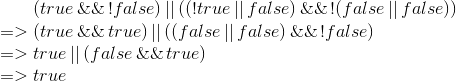All other combinations of values produce false.

### Example Question #4 : Boolean

#include <iostream>

using namespace std;
bool bigger(int x, int y)
{
if (x>y)
return true;
else
return false;
}
int main()
{

bool test;
test!=bigger(10,7);
cout<<test;
}

What will be the value of test after the previous code is run?

Possible Answers:

10

false

0

7

true

Correct answer:

0

Explanation:

The function "bigger" defined before main returns a value of type bool and takes in two integer inputs. It compares the first value to the second. If it it greater, it returns true. Otherwise, it returns false. In main, a new variable test is called, and it is of type bool as well. This automatically eliminates 3 out of the 5 possibilities.

"test" is defined as the opposite of the outcome of the bigger function with the two inputs are 10 and 7. 10 is bigger than 7, so the function returns true. Since test is the opposite of that, it is false.

### Example Question #2 : Boolean

Convert the decimal number 67 to binary.

Possible Answers:

1000010

1000101

1001010

1100011

1000011

Correct answer:

1000011

Explanation:

In a regular number system, also known as decimal, the position furtherest to the right is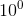, then one over to the left would be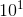, then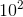. These are the ones, tens and hundreds place. Binary is a base 2 number system. Therefore, the digit to the furthest right is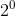, then to the left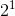, then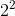, and so on.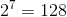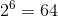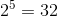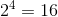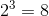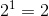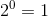Explanation

1000011  The bolded number has a value of 1

1000011  The bolded number has a value of 2

1000011  The bolded number has a value of 64

The positions that are marked true (as 1) in the binary number 1000011 corresponds to the numbers 64, 2, and 1 which added up equals 67

Step By Step

• To convert the number 67 to binary, first find the digit place number that has the largest number possible that is less than or equal to 67. In this case it would be the position holding• The number for 64 is 1000000
• To get 67, we need to add 3
• Again, find the digit place number that has the largest number possible less than or equal to 3. In this case, it would be• The number for 66 (64 + 2) is 1000010
• To get from 66 to 67, repeat the steps from before.
• The number for 67 (66 + 1) is 1000011

### All AP Computer Science A Resources